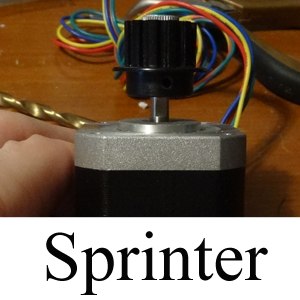Posted:4/3/2014 1:24AM

### Stepping Motor X/Y/Z/E Sprinter Firmware Calculator

A JavaScript based tool to calculate the 3d stepping motor X/Y/Z/E Sprinter firmware coefficients

From time to time my 3D printer begins to print rather inaccurately (typically deviating a few millimeters on either the X,Y, or Z axis) and I have to perform routine firmware calibration in order to bring the 3D printer back into proper alignment.

Likewise, while this particular process is rather straightforward and is also well documented (see the RepRap Triffid Hunter's Calibration Guide for more information); however, I typically run my printer off an older laptop that is somewhat unwieldy to operate and is not loaded down with convenient mathematical software (like Excel, MATLAB, et cetera).

Conversely, (with this being said) rather than muddling with the primitive calculator software provided by Windows, hobbling back and forth between the printer room and my master computer room (to run mathematical software), or trying to find my TI-89 calculator (noting that the batteries were dead when i did find it) I decided to write a simplistic JavaScript web application to perform the calibration calculations. Additionally, i also (for good measure) had my application output the calibration information in a format that could easily be copied into the Sprinter Firmware for easy compiling.

Noting that The predominant formula being utilized by this application is:

$S_{N}=S_{C}\left(\frac{D_{D}}{D_{M}}\right)$

where,

$S_{N}$

The New Sprinter Stepping Motor Value

$S_{C}$

The Current Sprinter Stepping Motor Value

$D_{D}$

The Desired Calibration Distance Moved

$D_{M}$

The Measured Distance Moved

and

$S_{N}=S_{C}\left(\frac{D_{D}}{D_{F}-D_{I}}\right)$

where,

$D_{I}$

An Initial Measurement From The Printer Frame To The Starting Location Being Measured

$D_{F}$

A Final Measurement From The Printer Frame To A Stopping Location Being Measured

Enjoy!